International
Tables for
Crystallography
Volume C
Mathematical, physical and chemical tables
Edited by E. Prince

International Tables for Crystallography (2006). Vol. C, ch. 7.5, pp. 666-667

## Section 7.5.3. Fixed-time counting

A. J. C. Wilsona

aSt John's College, Cambridge CB2 1TP, England

### 7.5.3. Fixed-time counting

| top | pdf |

In the absence of disturbing influences (mains-voltage fluctuations, unrectified or unsmoothed high-tension supplies, dead time' of the counter or counter circuits, etc.), the number of counts recorded during the predetermined time interval used in the fixed-time mode will fluctuate in accordance with the Poisson probability distribution. If the true' number of counts to be expected in the interval is N, the probability that the observed number will be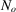is given by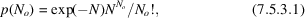where all quantities appearing are necessarily non-negative. Both the mean and the variance ofare N. If the true' number of counts to be expected when the diffractometer is set to receive a reflection is T, and the true' number when it is set to receive the immediate background is B, the true' intensity of the reflection is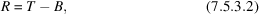provided that the time interval used for the reflection is equal to the time interval used for the background. In practice, the observed' values ofand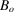fluctuate with probabilities given by (7.5.3.1)with T or B replacing N, so that the observed value,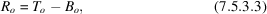will also fluctuate, and can, occasionally, take on negative values. The treatment of `measured-as-negative' intensities is discussed in Section 7.5.6.

Although the sum of two Poisson-distributed variables is also Poisson, the difference is not, and the probability of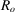given by (7.5.3.3)has been shown to be (Skellam, 1946; Wilson, 1978)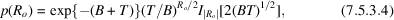whereis the hyperbolic Bessel function of the first kind. The mean and variance ofare TB and T + B, respectively. If the times used for reflection and background are not equal, but are in the ratio of k : 1, the mean and variance of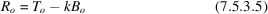are TkB and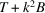, respectively. The distribution ofthen involves a generalized Bessel function1 depending on k: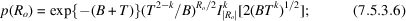for the properties of the generalized Bessel function, see Wright (1933) and Olkha & Rathie (1971). The probability distributions (7.5.3.4)and (7.5.3.6)seem to be very close to a normal distribution with the same mean and variance fornear R, but the probability of moderate deviations from R is less than normal and for large deviations is greater than normal.

In applications, it is usual to work with intensities expressed as counting rates, rather than as numbers of counts, even when fixed-time counting is used. The total intensity τ expressed as a counting rate is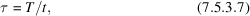where t is the time devoted to the measurement, and the variance of the counting rate is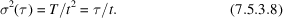Similar expressions apply for the background, with B for the count, b for the time, and β for the counting rate. For the reflection count, the corresponding expressions are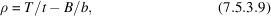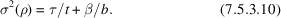To avoid confusion, upper-case italic letters are used for numbers of counts, lower-case italic for counting times, and the corresponding lower-case Greek letters for the corresponding counting rates. In accordance with common practice, however,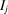will be used for the intensity of the jth reflection, the context making it clear whether I is a number of counts or a counting rate.

### References

Olkha, G. S. & Rathie, P. N. (1971). On a generalized Bessel function and an integral transform. Math. Nachr. 51, 231–240.
Skellam, J. G. (1946). The frequency distribution of the difference between two Poisson values belonging to different populations. J. R. Stat. Soc. 109, 296.
Wilson, A. J. C. (1978). On the probability of measuring the intensity of a reflection as negative. Acta Cryst. A34, 474–475.
Wright, E. M. (1933). On the coefficients of power series having exponential singularities. J. London Math. Soc. 8, 71–79.